• 32
•
•
•
•
•
•
•
32
Shares

# Continuity of a function

we had studied about limit of a function at a point now we will study about the continuity of a function, what does it means for a function to be continuous at a point ? how we can find out whether a function is continuous or not ? How to know whether a composite function is continuous or not.

## Continuity

### Continuous and Discontinuous Functions

In Simple meaning we say a function y=f(x)  is a continuous function if we can draw the graph of the function without lifting pen from paper . But if while drawing graph of a function if we have to lift pen from paper atleast one time then we say the function is discontinuous, a discontinuous function will have break on its graph

the above image illustrates a discontinuous Function followed by a continuous function and at last a discontinuous Function.

## Mathematical Definition For Continuity

#### Continuity At a Point

Any function y=f(x) is defined to be a continuous function at x=a if the graph of the function doesn’t break around x=a, Mathematically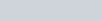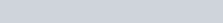if this isn’t satisfied by the function then the function will be discontinuous.

#### Continuity In an Interval

1.Open Interval – Any function y=f(x) is defined to be continuous in open interval,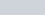if the function is continuous at every point in the same interval. if not then the function would be discontinuous in the interval discussed.

2.Closed Interval– Any function y=f(x) is defined to be continuous in closed interval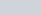if it satisfies the following conditions-

 (i) f(x) is continuous in(ii) f(a) =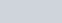(iii)f(b) =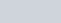If the above conditions aren’t satisfied the function would be discontinuous.

 POINT TO BE NOTED: • Continuity of the function Should be checked at –• Points( value of x) where the behavior/ Definition of the function Changes .• For Signum ( sgn f(x) ) Function we also check continuity where the value of the function vanishes i.e f(x)= 0• For Greatest Integer function and fractional part function we check the continuity at the points where f(x)= integer. i.e where the value of function becomes integer2. Its noteworthy that all Polynomial Functions, Logarithmic Functions, Trigonometric Functions,  Inverse trigonometric functions and Exponential Functions are Continuous in Their respective domains , it can be seen from the curve of these functions.

## Theorems On Continuity

1. if f(x) and g(x) are continuous at x=a then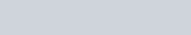all will be continuous at x=a.

2. if f(x) is continuous and g(x) is discontinuous at x=a then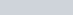will be discontinuous at x=a , and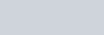may be continuous or may be discontinuous at x=a.

3.if f(x) and g(x) are discontinuous at x=a thenmay be continuous at x=a.

4. For Composite Functions , if f(x) is continuous at x=c and g(x) is continuous at x=f(c) then gof(x) will be continuous at x=c. it’s advised to check continuity of gof(x) at points where f(x) is discontinuous.

## Intermediate Value theorem

The intermediate value theorem is an important theorem Regarding continuous functions. Intermediate value theorem is of great importance in Mathematics, particularly  in functional analysis. Actually this theorem shows the application of concept of continuity of a function which we’d studied.

Talking for a continuous function y=f(x) in a closed intervalintermediate value theorem states that

1.  if f(a) and f(b) are of opposite signs then there exists atleast one solution for the equation f(x)=0. in the intervalit can be visualized and understood by the figure shown below.

2. if “K” is any real no. such that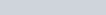then there exists atleast one solution of the equation f(x)= K. this can be interpreted from the above figure.

# Discontinuity

If any function is not continuous (at x=a) as per definition of continuity, then the function is called as discontinuous. The point x=a is called as point of discontinuity.

## Types of Discontinuity

Here, Broadly we’re discussing following types of Discontinuity as summarised below-

 1. Removable Discontinuity2. Discontinuity of First Kind (Ordinary Discontinuity)3. Discontinuity of Second Kind.4. Mixed Discontinuity5. Infinite Discontinuity6. Oscillating Discontinuity

#### Removable Discontinuity

Talking for graph of a function at a point x=a if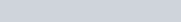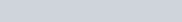and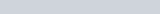But,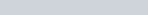This kind of Discontinuity is known as Removable Discontinuity as illustrated in image also.

#### Discontinuity of First Kind (ordinary discontinuity )

Talking for graph of a function at a point x=a  ifBut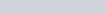Here at max any one of the L.H.L or R.H.L can may be equal to f(a).
This type of discontinuity is known as discontinuity of First Kind.

#### Discontinuity of Second Kind

Talking for graph of a function at a point x=a  if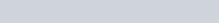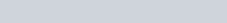If L.H.L and R.H.L Both doesn’t exist at a point then it’s called as Discontinuity of Second Kind.

#### Mixed Discontinuity

If any one of the L.H.L or R.H.l is of first kind (exists) and the other is if second kind (doesn’t exists ), this discontinuity is referred as Mixed Discontinuity.

#### Infinite Discontinuity

If the limits L.H.L Reaches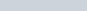and Also R.H.L reaches any ofThis type of discontinuity is known as Infinite Discontinuity.

#### Oscillating Discontinuity

Any function is defined to be having an oscillating Discontinuity at x=a if the L.H.L and R.H.L lies in a certain range but its value doesn’t approaches to a particular value at x=a. It seems that the limits are approaching to two or more values.

For example- take a look at the curve of sin(1/x) as x goes near zero the value of 1/x becomes very large but not any finite no. Also the range of sine function is [-1,1]. Hence the value of function oscillates between [-1,1]. This discontinuity is Oscillating Discontinuity.

Grasped Concepts of Continuity ? Time to Understand Differentiability of a Function.